Skip to main content
Equity. Access. Excellence.

# DCMP Statistical Analysis Tools

Explore statistical concepts in an interactive way. Use the DCMP Statistical Analysis Tools to construct graphs, obtain summary statistics, find probabilities under the normal distribution, get confidence intervals, fit linear regression models, and more!

The Dana Center Mathematics Pathways (DCMP) offers this suite of statistical analysis tools to  complement the Dana Center’s Introductory Statistics: Analyzing Data with Purpose course.

Throughout this course, students analyze data, construct and test hypotheses, solve problems, reflect on their work, and make connections between concepts. Students use the DCMP Statistical Analysis Tools to analyze and understand data and run simulations to promote deep understanding of statistical analysis.

## Exploratory Data Analysis

• Describing and Exploring Categorical Data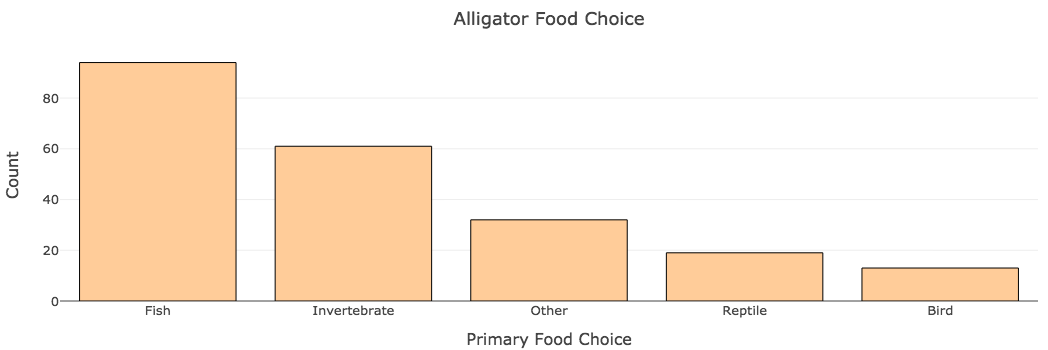Construct frequency and contingency tables and bar graphs to explore distributions of categorical variables. For one or two categorical variables.

• Describing and Exploring Quantitative DataFind summary statistics and construct interactive histograms, boxplots, dotplots, or stem-and leaf plots. For one, two, or more groups.

• Explore Time Series Data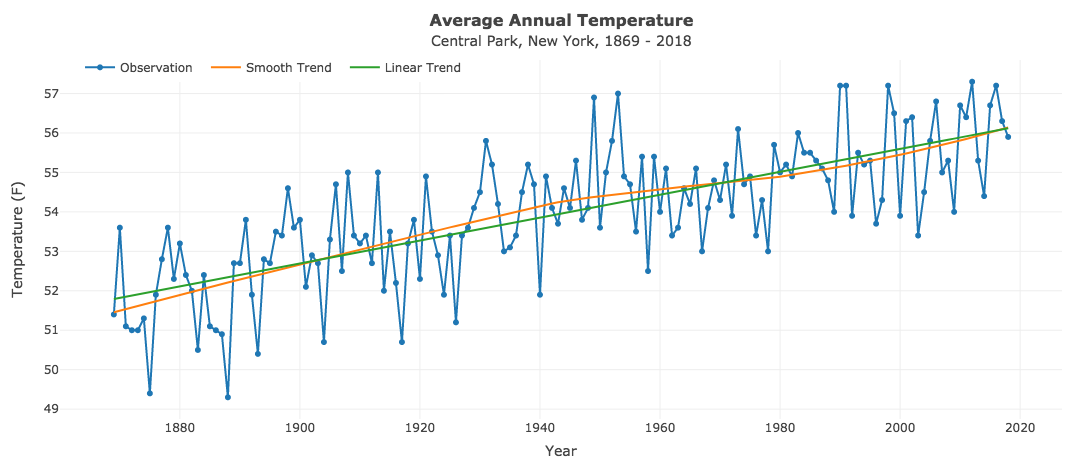Plot a simple time series and add a smooth or linear trend. Use preloaded data or provide your own.

• Mean vs. Median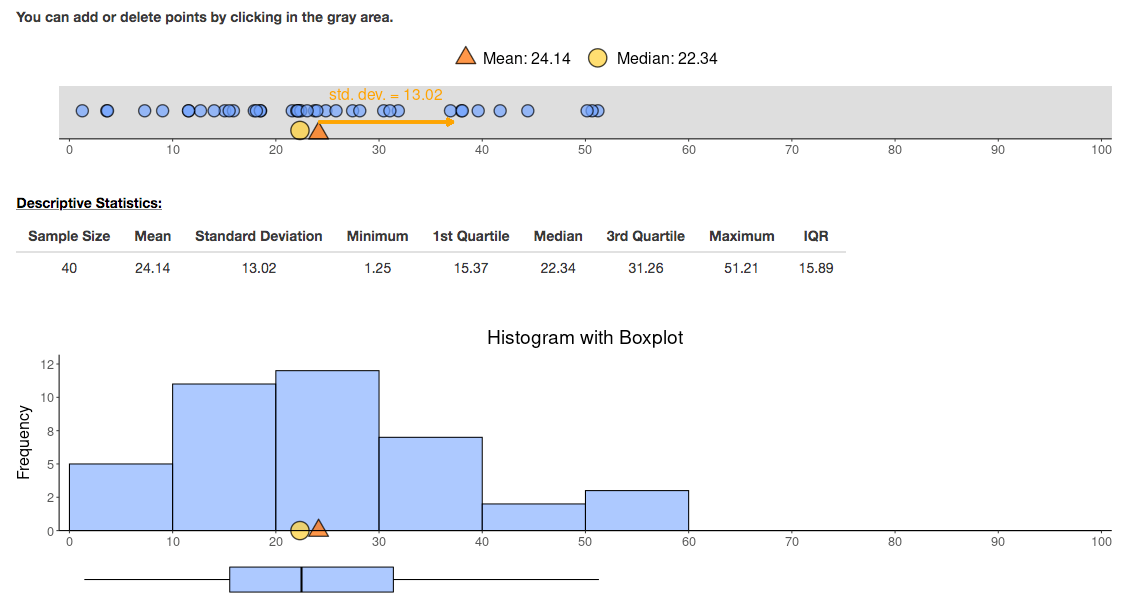Explore the relationship between the mean and median for data derived from a variety of distributions or enter your own data.

• Generate Random Numbers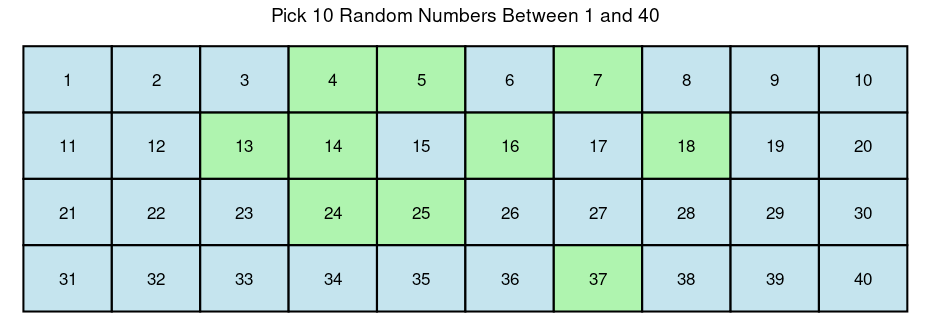Generate random numbers or flips of a (biased) coin. Keep track of generated numbers with a bar chart.

## Association, Correlation, and Regression

• Association Between Two Categorical Variables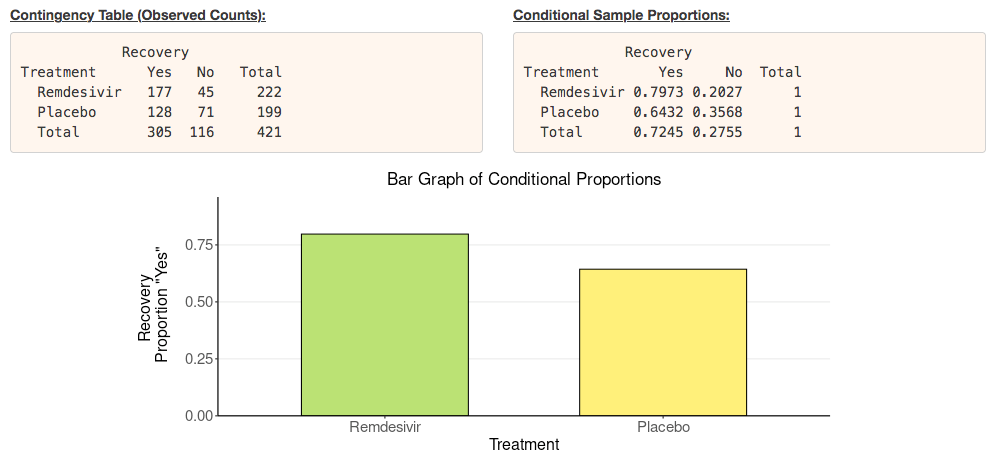Construct 2 x 2 contingency tables, obtain conditional proportions, and get a bar graph. Find the difference or ratio of proportions to describe the strength of the association. Build the sampling distribution of the difference or ratio via resampling.

• Relationship Between Two Categorical Variables: The Correlation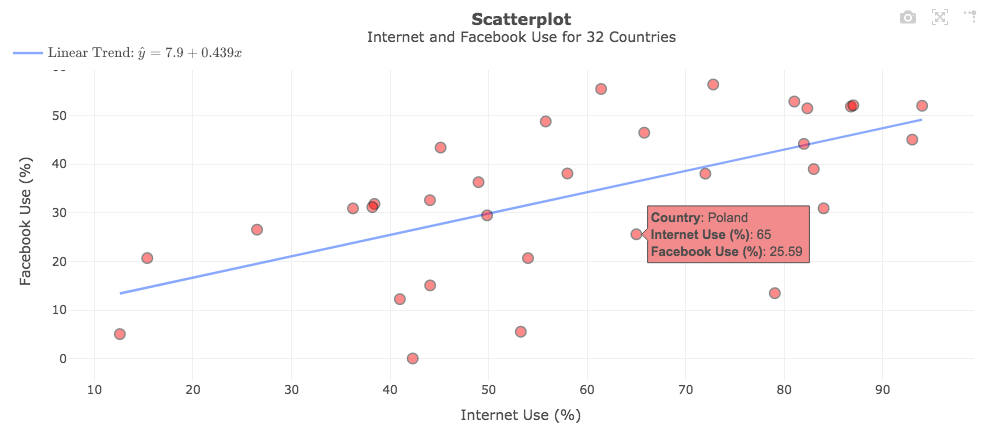Construct interactive scatterplots, hover over points, move or remove points, and overlay a smooth trend line. Find the correlation coefficient r and see if it is robust to outliers. Build the sampling distribution of r via resampling.

• Correlation Game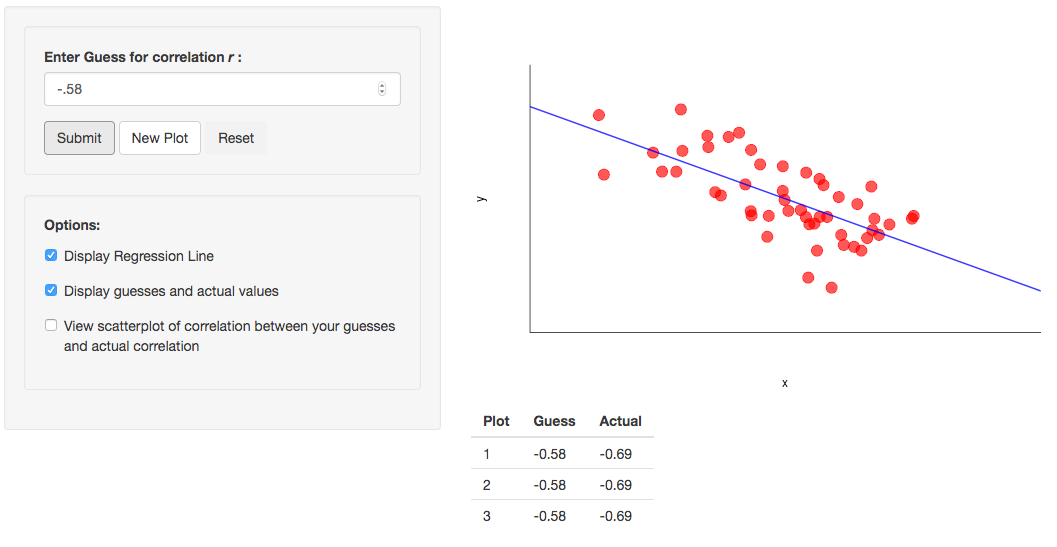Randomly generate scatterplots to guess the correlation coefficient r. Optionally, display the regression line. See how your guesses correlate with the actual values.

• Explore Linear Regression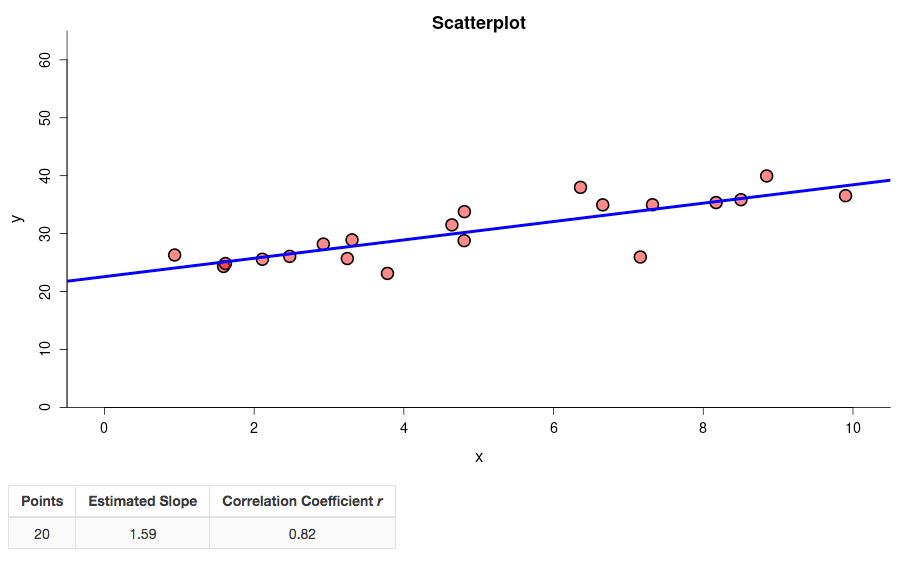Create scatterplots from scratch by clicking in an empty plot to add or remove points. Investigate the effect of outliers on the correlation coefficient or regression line. Simulate linear or non-linear relationships.

• Linear Regression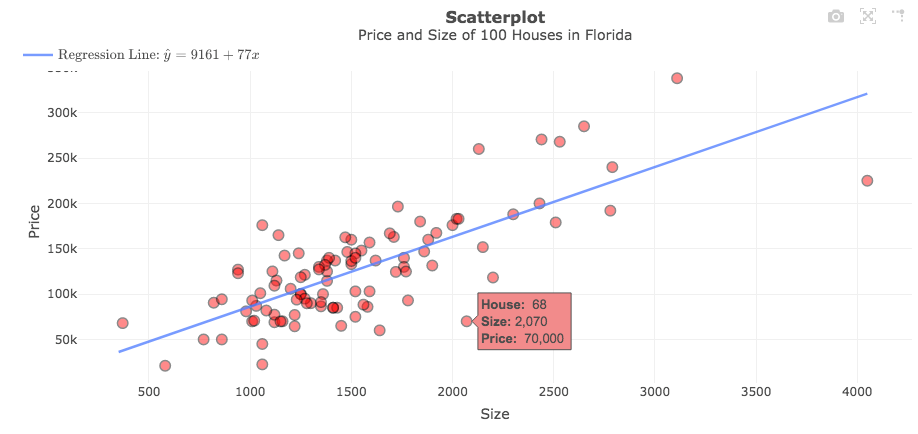Fit a simple linear regression model and obtain the regression equation and related statistics such as r-squared. Make predictions and construct confidence intervals. Display and analyze residuals.

• Multivariate Relationships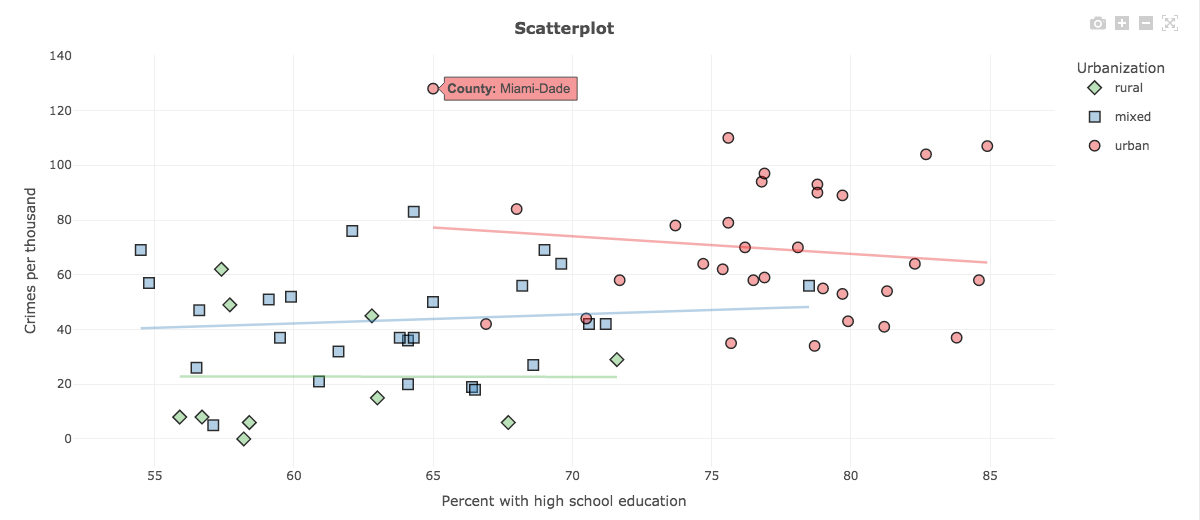Construct interactive scatterplots to explore the relationship between two quantitative variables while accounting for a third (categorical or quantitative) grouping variable. Fit bivariate multiple linear regression models.

## Distributions

• The Normal Distribution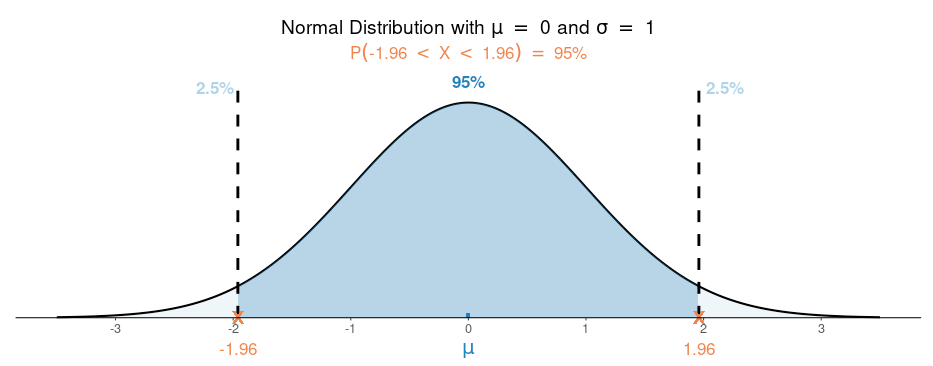See how the shape of the normal distribution depends on the mean and standard deviation. Find and visualize one- and two-tailed probabilities and percentiles (critical values).

• The t Distribution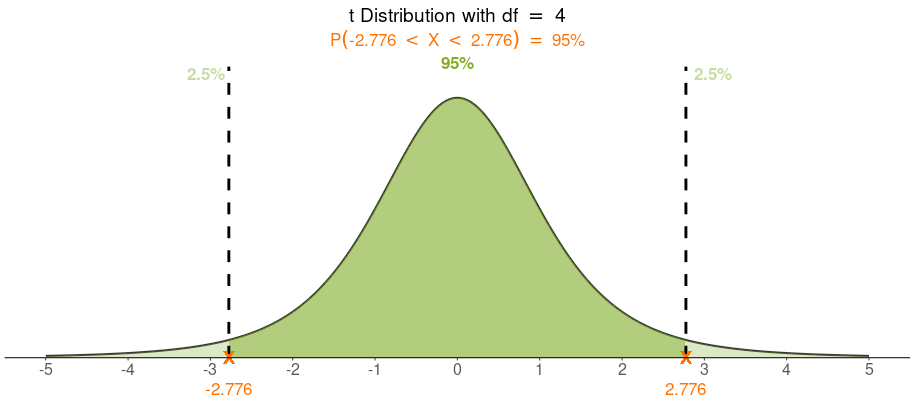See how the shape of the t distribution depends on the degrees of freedom. Find and visualize one- and two-tailed probabilities and percentiles (critical values).

• The Chi-Squared Distribution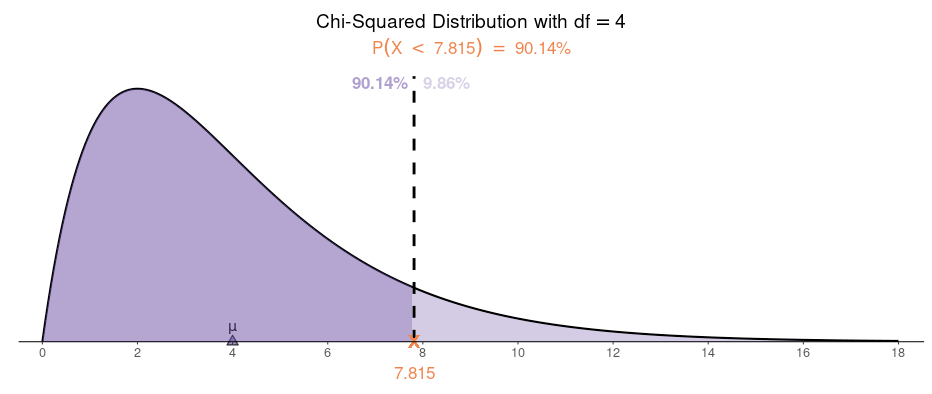See how the shape of the chi-squared distribution depends on the degrees of freedom. Find and visualize probabilities and percentiles (critical values).

• The F Distribution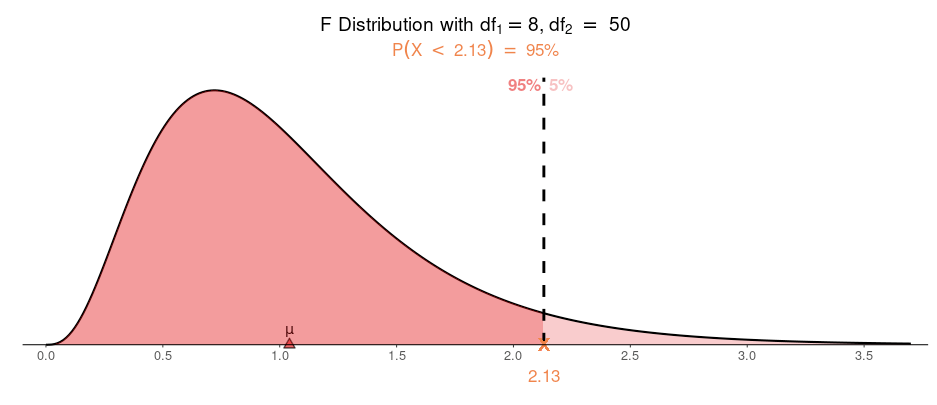See how the shape of the F distribution depends on the degrees of freedom. Find and visualize probabilities and percentiles (critical values).

• The Binomial Distribution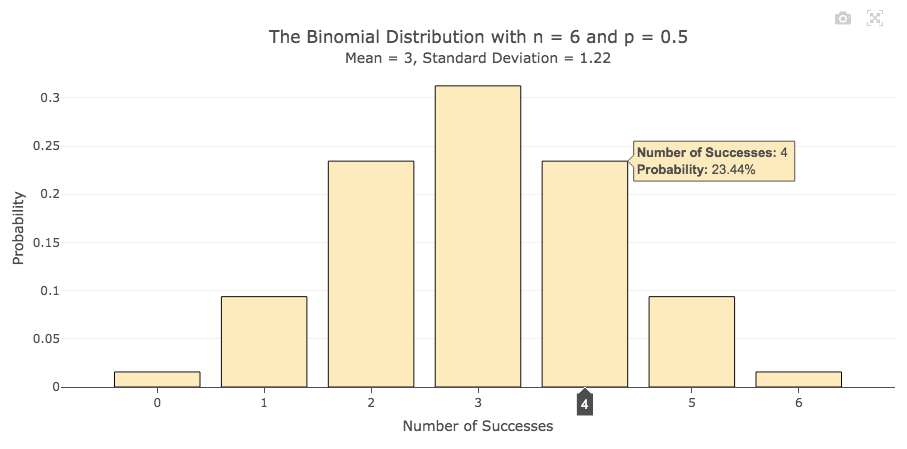Explore how the shape of the binomial distribution depends on the parameter n (the sample size) and p (the probability of success in a Bernoulli trial). Find and visualize probabilities of various kinds.

• The Poisson Distribution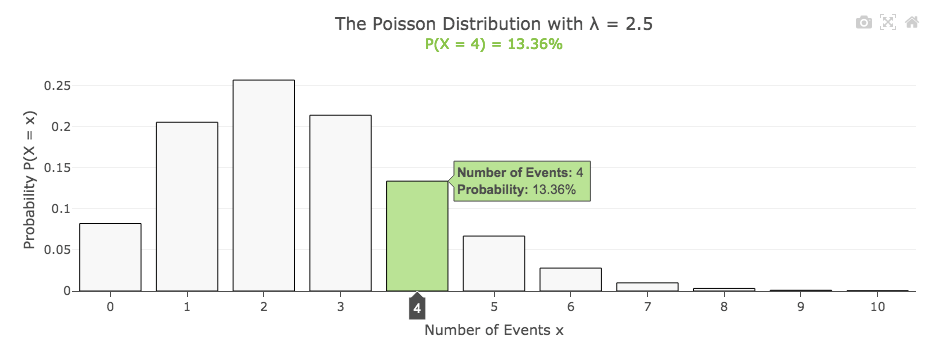Explore how the shape of the Poisson distribution depends on the parameter λ (the mean). Find and visualize various kinds of probabilities.